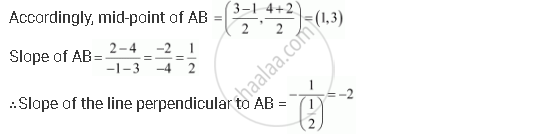CBSE (Arts) Class 11CBSE
Share

# Find the Equation of the Right Bisector of the Line Segment Joining the Points (3, 4) and (–1, 2). - CBSE (Arts) Class 11 - Mathematics

ConceptGeneral Equation of a Line

#### Question

Find the equation of the right bisector of the line segment joining the points (3, 4) and (1, 2).

#### Solution

The right bisector of a line segment bisects the line segment at 90°.

The end-points of the line segment are given as A (3, 4) and B (–1, 2).The equation of the line passing through (1, 3) and having a slope of –2 is

(y – 3) = –2 (x – 1)

y – 3 = –2x + 2

2x + y = 5

Thus, the required equation of the line is 2x + y = 5.

Is there an error in this question or solution?

#### Video TutorialsVIEW ALL 

Solution Find the Equation of the Right Bisector of the Line Segment Joining the Points (3, 4) and (–1, 2). Concept: General Equation of a Line.
S